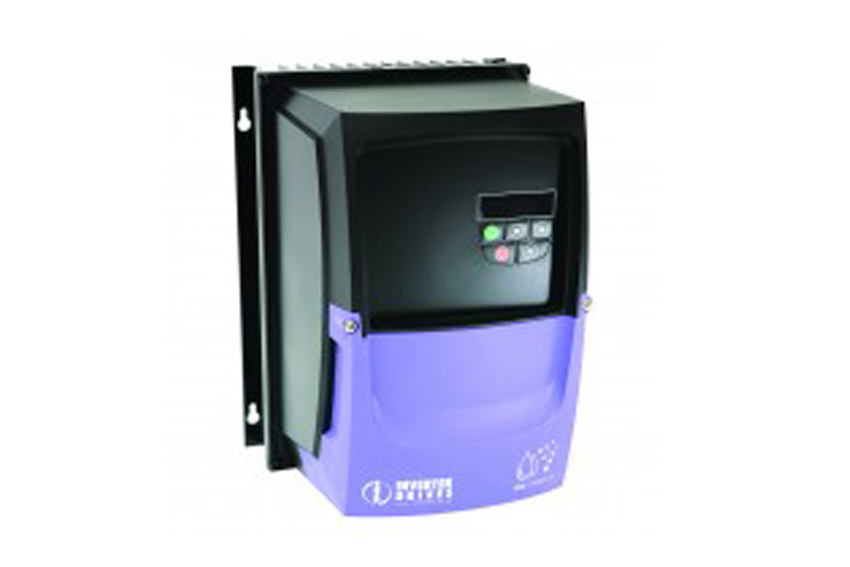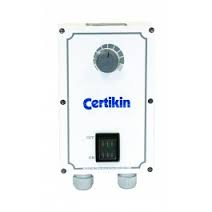# Inverters/Variable Speed Drives

This variable speed inverter offers a potential payback after only six months. Easy to install and programme, it is suitable for various pump models and can be linked to a building management system.

### Full Description

EXAMPLE OF COST SAVING:

Pump Size - 5.5hp (4.1kW) 3 phase pump

Operating Time - 24hrs per day/ 7 days a week/ 52 weeks a year

Cost of Unit - £605.00

Cost of Installation - £305.00

Total Cost - £955.00

Based on electric at 11p per kWh the cost saving running the unit at 80% of the speed for 95% of time and 100% of speed for 5% (backwash). Cost saving is £98.00 per months payback on  installation.

Understanding pump affinity laws will allow you to explain, demonstrate and calculate how a change in motor speed has an impact:

- Flow rates against resistance

- Power consumption and operating costs

1. The change in flow is proportionate (equal) to the change in speed

- if we halve the speed we halve the flow

2. The change in pressure is equal to the speed change factor squared

-  Operating pressure will be 1/4 of the original pressure

3. The change in power consumption is equal to the speed change factor cubed

- Power consumption will be 1/8 the original consumption

As an example if we take a pump with the following characteristics:

- Motor Speed - 2850rpm

- Flow Rate - 302 Ipm

- Pressure - 8 metres head

- Power input - 983 Watts

We set the low speed at 1900 rpm - a reduction factor of 0.67

- 1900 divided by 2850 = 0.67

- Applying the factor 0.67 to all pump affinity laws we can determine the pumps operating characteristics at the new speed on 1900rpm

1. The change in flow is proportionate (equal) to the change in speed

- 302 x 0.67 = 202 Ipm

2. The change in pressure is equal to the speed change factor squared

- 8 x 0.67 x 0.67 = 3.6 meters head

3. The change in power consumption is equal to the speed change factor cubed

- 983 x 0.67 x 0.67 x 0.67 = 295 Watts

Therefore the pump has these new low speed operating characteristics

- Motor Speed - 1900 rpm

- Flow Rate 202 - Ipm

- Pressure 3.6 - meters head

- Power Input - 295 Watts